| Table of Contents | Function Reference | Function Finder | R Project | SIMPLE LINEAR CORRELATION AND REGRESSION Correlation Correlation is used to test for a relationship between two numeric variables or two ranked (ordinal) variables. In this tutorial, we assume the relationship (if any) is linear. To demonstrate, we will begin with a data set called "cats" from the "MASS" library, which contains information on various anatomical features of house cats. ```> library("MASS") > data(cats) > str(cats) 'data.frame': 144 obs. of 3 variables: \$ Sex: Factor w/ 2 levels "F","M": 1 1 1 1 1 1 1 1 1 1 ... \$ Bwt: num 2 2 2 2.1 2.1 2.1 2.1 2.1 2.1 2.1 ... \$ Hwt: num 7 7.4 9.5 7.2 7.3 7.6 8.1 8.2 8.3 8.5 ... > summary(cats) Sex Bwt Hwt F:47 Min. :2.000 Min. : 6.30 M:97 1st Qu.:2.300 1st Qu.: 8.95 Median :2.700 Median :10.10 Mean :2.724 Mean :10.63 3rd Qu.:3.025 3rd Qu.:12.12 Max. :3.900 Max. :20.50``` "Bwt" is the body weight in kilograms, "Hwt" is the heart weight in grams, and "Sex" should be obvious. There are no missing values in any of the variables, so we are ready to begin by looking at a scatterplot. ```> with(cats, plot(Bwt, Hwt)) > title(main="Heart Weight (g) vs. Body Weight (kg)\nof Domestic Cats")```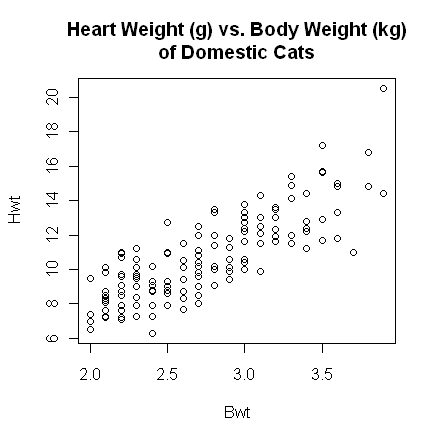The plot() function gives a scatterplot whenever you feed it two numeric variables. The first variable listed will be plotted on the horizontal axis. A formula interface can also be used, in which case the response variable should come before the tilde and the variable to be plotted on the horizontal axis after. (Close the graphics window before doing this, because the output will look exactly the same.) ```> with(cats, plot(Hwt ~ Bwt)) # or... > plot(Hwt ~ Bwt, data=cats)``` The scatterplot shows a fairly strong and reasonably linear relationship between the two variables. A Pearson product-moment correlation coefficient can be calculated using the cor() function (which will fail if there are missing values). ```> with(cats, cor(Bwt, Hwt))  0.8041274 > with(cats, cor(Bwt, Hwt))^2  0.6466209``` Pearson's r = .804 indicates a strong positive relationship. To get the coefficient of determination, I just hit the up arrow key to recall the previous command to the command line and added "^2" on the end to square it. If a test of significance is required, this is also easily enough done using the cor.test() function. The function does a t-test, a 95% confidence interval for the population correlation (use "conf.level=" to change the confidence level), and reports the value of the sample statistic. The alternative hypothesis can be set to "two.sided" (the default), "less", or "greater". (Meaning less than 0 or greater than 0 in most cases.) ```> with(cats, cor.test(Bwt, Hwt)) # cor.test(~Bwt + Hwt, data=cats) also works Pearson's product-moment correlation data: Bwt and Hwt t = 16.1194, df = 142, p-value < 2.2e-16 alternative hypothesis: true correlation is not equal to 0 95 percent confidence interval: 0.7375682 0.8552122 sample estimates: cor 0.8041274``` Since we would expect a positive correlation here, we might have set the alternative to "greater". ```> with(cats, cor.test(Bwt, Hwt, alternative="greater", conf.level=.8)) Pearson's product-moment correlation data: Bwt and Hwt t = 16.1194, df = 142, p-value < 2.2e-16 alternative hypothesis: true correlation is greater than 0 80 percent confidence interval: 0.7776141 1.0000000 sample estimates: cor 0.8041274``` There is also a formula interface for cor.test(), but it's tricky. Both variables should be listed after the tilde. (Order of the variables doesn't matter in either the cor() or the cor.test() function.) `> cor.test(~ Bwt + Hwt, data=cats) # output not shown` Using the formula interface makes it easy to subset the data by rows of the data frame. ```> cor.test(~Bwt + Hwt, data=cats, subset=(Sex=="F")) Pearson's product-moment correlation data: Bwt and Hwt t = 4.2152, df = 45, p-value = 0.0001186 alternative hypothesis: true correlation is not equal to 0 95 percent confidence interval: 0.2890452 0.7106399 sample estimates: cor 0.5320497``` The "subset=" option is not available unless you use the formula interface. For a more revealing scatterplot, try this. ```> with(cats, plot(Bwt, Hwt, type="n", las=1, xlab="Body Weight in kg", + ylab="Heart Weight in g", + main="Heart Weight vs. Body Weight of Cats")) > with(cats, points(Bwt[Sex=="F"], Hwt[Sex=="F"], pch=16, col="red")) > with(cats, points(Bwt[Sex=="M"], Hwt[Sex=="M"], pch=17, col="blue"))```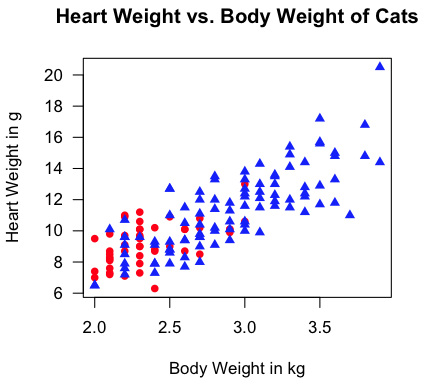"Aw, man! I'm not gonna type all that!" Fine! I don't blame you (much). Copy those lines from this webpage and then paste them into a script window (File > New Script in Windows or File > New Document on a Mac). Erase the command prompts, and then execute. But if you type it yourself, you get to see what happens step by step. (See the tutorial on scripts for details on how to use them.) Correlation and Covariance Matrices If a data frame (or other table-like object) contains more than two numeric variables, then the cor() function will result in a correlation matrix. ```> rm(cats) # if you haven't already > data(cement) # also in the MASS library > str(cement) 'data.frame': 13 obs. of 5 variables: \$ x1: int 7 1 11 11 7 11 3 1 2 21 ... \$ x2: int 26 29 56 31 52 55 71 31 54 47 ... \$ x3: int 6 15 8 8 6 9 17 22 18 4 ... \$ x4: int 60 52 20 47 33 22 6 44 22 26 ... \$ y : num 78.5 74.3 104.3 87.6 95.9 ... > cor(cement) # fails if there are missing values x1 x2 x3 x4 y x1 1.0000000 0.2285795 -0.82413376 -0.24544511 0.7307175 x2 0.2285795 1.0000000 -0.13924238 -0.97295500 0.8162526 x3 -0.8241338 -0.1392424 1.00000000 0.02953700 -0.5346707 x4 -0.2454451 -0.9729550 0.02953700 1.00000000 -0.8213050 y 0.7307175 0.8162526 -0.53467068 -0.82130504 1.0000000``` If you prefer a covariance matrix, use cov(). ```> cov(cement) # also fails if there are missing values x1 x2 x3 x4 y x1 34.60256 20.92308 -31.051282 -24.166667 64.66346 x2 20.92308 242.14103 -13.878205 -253.416667 191.07949 x3 -31.05128 -13.87821 41.025641 3.166667 -51.51923 x4 -24.16667 -253.41667 3.166667 280.166667 -206.80833 y 64.66346 191.07949 -51.519231 -206.808333 226.31359``` If you have a covariance matrix and want a correlation matrix... ```> cov.matr = cov(cement) > cov2cor(cov.matr) x1 x2 x3 x4 y x1 1.0000000 0.2285795 -0.82413376 -0.24544511 0.7307175 x2 0.2285795 1.0000000 -0.13924238 -0.97295500 0.8162526 x3 -0.8241338 -0.1392424 1.00000000 0.02953700 -0.5346707 x4 -0.2454451 -0.9729550 0.02953700 1.00000000 -0.8213050 y 0.7307175 0.8162526 -0.53467068 -0.82130504 1.0000000``` If you want a visual representation of the correlation matrix (i.e., a scatterplot matrix)... `> pairs(cement)`The command plot(cement) would also have done the same thing. Correlations for Ranked Data If the data are ordinal rather than true numerical measures, or have been converted to ranks to fix some problem with distribution or curvilinearity, then R can calculate a Spearman rho coefficient or a Kendall tau coefficient. Suppose we have two athletic coaches ranking players by skill. ```> ls()  "cement" "cov.matr" > rm(cement, cov.matr) # clean up first > coach1 = c(1,2,3,4,5,6,7,8,9,10) > coach2 = c(4,8,1,5,9,2,10,7,3,6) > cor(coach1, coach2, method="spearman") # do not capitalize "spearman"  0.1272727 > cor.test(coach1, coach2, method="spearman") Spearman's rank correlation rho data: coach1 and coach2 S = 144, p-value = 0.72 alternative hypothesis: true rho is not equal to 0 sample estimates: rho 0.1272727 > cor(coach1, coach2, method="kendall")  0.1111111 > cor.test(coach1, coach2, method="kendall") Kendall's rank correlation tau data: coach1 and coach2 T = 25, p-value = 0.7275 alternative hypothesis: true tau is not equal to 0 sample estimates: tau 0.1111111 > ls()  "coach1" "coach2" > rm(coach1,coach2) # clean up again``` I will not get into the debate over which of these rank correlations is better! You can also use the "alternative=" option here to set a directional test. How To Get These Functions To Work If There Are Missing Values As noted, missing values cause these functions to cough up a hair ball. Here's how to get around it. We'll use the cats data again, but will insert a few missings. ```> data(cats) > cats[12,2] = NA > cats[101,3] = NA > cats[132,2:3] = NA > summary(cats) Sex Bwt Hwt F:47 Min. :2.000 Min. : 6.30 M:97 1st Qu.:2.300 1st Qu.: 8.85 Median :2.700 Median :10.10 Mean :2.723 Mean :10.62 3rd Qu.:3.000 3rd Qu.:12.07 Max. :3.900 Max. :20.50 NA's :2 NA's :2 > with(cats, cor(Bwt, Hwt))  NA``` Okay, it's not a hair ball, it's an NA. Easier on your carpet! Let's see what works and what doesn't. ```> with(cats, cor(Bwt, Hwt)) # fails > with(cats, cov(Bwt, Hwt)) # fails > with(cats, cor.test(Bwt, Hwt)) # works! (I didn't think it would.) > with(cats, plot(Bwt, Hwt)) # works``` To get cor() and cov() to work with missing values, you set the "use=" option. Possible values are "everything", "all.obs" (not sure what the difference is there), "complete.obs" (deletes cases with any missing values from the computation even if there are no missings in the two variables that are currently being computed with), "na.or.complete" (pretty much the same), and "pairwise.complete.obs" (all complete pairs of the two variables currently being considered are used). ```> with(cats, cor(Bwt, Hwt, use="pairwise"))  0.8066677``` You only need to type enough of the option value that R can recognize it (thank goodness in this case). Simple Linear Regression There is little question that R was written with regression analysis in mind! The number of functions and add-on packages devoted to regression analysis of one kind or another is staggering! We will only scratch the surface in this tutorial. Let's go back to our kitty cat data. ```> rm(cats) # get rid of the messed up version > data(cats) # still in the "MASS" library``` Simple (as well as multiple) linear regression is done using the lm() function. This function requires a formula interface, and for simple regression the formula takes the form DV ~ IV, which should be read something like "DV as a function of IV" or "DV as modeled by IV" or "DV as predicted by IV", etc. It's critical to remember that the DV or response variable must come before the tilde and IV or explanatory variables after. Getting the regression equation for our "cats" is simple enough. ```> lm(Hwt ~ Bwt, data=cats) Call: lm(formula = Hwt ~ Bwt, data = cats) Coefficients: (Intercept) Bwt -0.3567 4.0341``` So the regression equation is: Hwt-hat = 4.0341 (Bwt) - 0.3567. A better idea is to store the output into a data object and then to extract information from that with various extractor functions. ```> lm.out = lm(Hwt ~ Bwt, data=cats) # name the output anything you like > lm.out # gives the default output Call: lm(formula = Hwt ~ Bwt, data = cats) Coefficients: (Intercept) Bwt -0.3567 4.0341 > summary(lm.out) # gives a lot more info Call: lm(formula = Hwt ~ Bwt, data = cats) Residuals: Min 1Q Median 3Q Max -3.5694 -0.9634 -0.0921 1.0426 5.1238 Coefficients: Estimate Std. Error t value Pr(>|t|) (Intercept) -0.3567 0.6923 -0.515 0.607 Bwt 4.0341 0.2503 16.119 <2e-16 *** --- Signif. codes: 0 '***' 0.001 '**' 0.01 '*' 0.05 '.' 0.1 ' ' 1 Residual standard error: 1.452 on 142 degrees of freedom Multiple R-squared: 0.6466, Adjusted R-squared: 0.6441 F-statistic: 259.8 on 1 and 142 DF, p-value: < 2.2e-16``` Personally, I don't like those significance stars, so I'm going to turn them off. (It'll also save me from having to erase the smart quotes from the R output and replace them with ordinary sane quotes. Really, R people? Smart quotes?!) ```> options(show.signif.stars=F) > anova(lm.out) # shows an ANOVA table Analysis of Variance Table Response: Hwt Df Sum Sq Mean Sq F value Pr(>F) Bwt 1 548.09 548.09 259.83 < 2.2e-16 Residuals 142 299.53 2.11```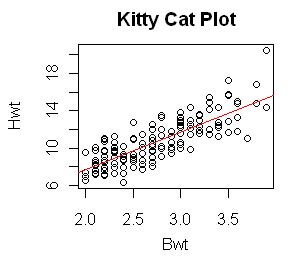With the output saved in a data object, plotting the regression line on a pre-existing scatterplot is a cinch. ```> plot(Hwt ~ Bwt, data=cats, main="Kitty Cat Plot") > abline(lm.out, col="red")``` And I'll make the regression line red just because I can! Various regression diagnostics are also available. These will be discussed more completely in future tutorials, but for now, a graphic display of how good the model fit is can be achieved as follows. ```> par(mfrow=c(2,2)) # partition the graphics device > plot(lm.out)```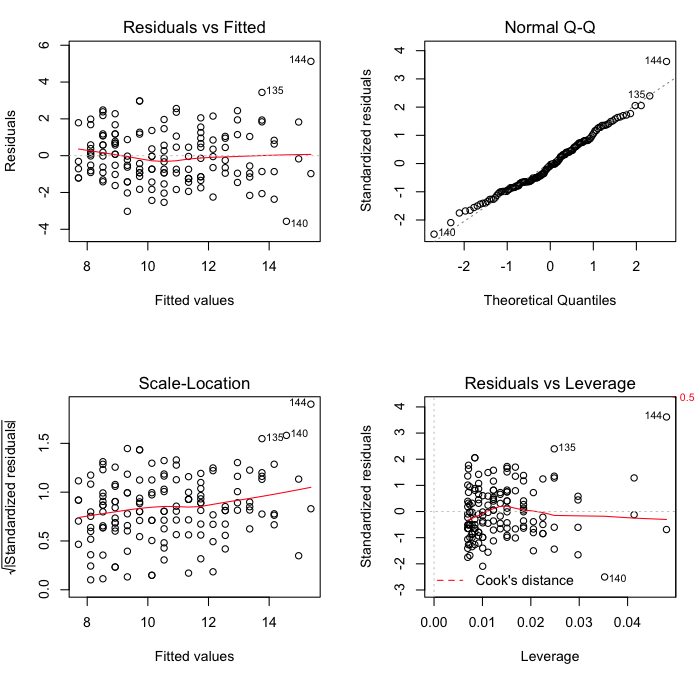The first of these commands sets the parameters of the graphics device. If your graphics output window was closed, you probably noticed it opening when this command was issued. Specifically, the command partitioned the graphics window into four parts, two rows by two columns, so four graphs could be plotted in one window. The graphics window will retain this structure until you either close it, in which case it will return to its default next time it opens, or you reset it with par(mfrow=c(1,1)). If you do not partition the graphics window first, then each diagnostic plot will print out in a separate window. R will give you a cryptic message, "Waiting to confirm page change...", in the console before each graph is displayed. This means hit the Enter key to display the next graph. The second command, plot(), then does the actual plotting. The first plot is a standard residual plot showing residuals against fitted values. Points that tend towards being outliers are labeled. If any pattern is apparent in the points on this plot, then the linear model may not be the appropriate one. The second plot is a normal quantile plot of the residuals. We like to see our residuals normally distributed. Don't we? The last plot shows residuals vs. leverage. Labeled points on this plot represent cases we may want to investigate as possibly having undue influence on the regression relationship. Case 144 is one perhaps worth taking a look at. ```> cats[144,] Sex Bwt Hwt 144 M 3.9 20.5 > lm.out\$fitted 144 15.37618 > lm.out\$residuals 144 5.123818``` This cat had the largest body weight and the largest heart weight in the data set. (See the summary table at the top of this tutorial.) The observed value of "Hwt" was 20.5g, but the fitted value was only 15.4g, giving a residual of 5.1g. The residual standard error (from the model output above) was 1.452, so converting this to a standardized residual gives 5.124/1.452 = 3.53, a substantial value to say the least! A commonly used measure of influence is Cook's Distance, which can be visualized for all the cases in the model as follows. ```> par(mfrow=c(1,1)) # if you haven't already done this > plot(cooks.distance(lm.out)) # also try plot(lm.out, 4)```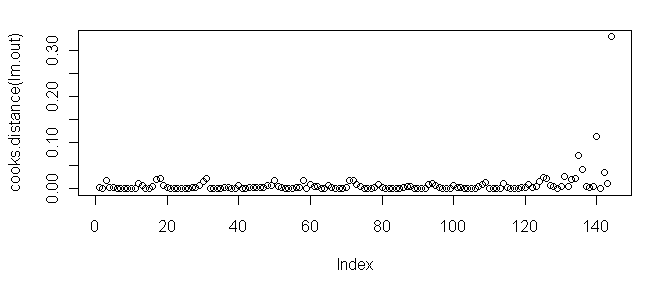As we can see, case 144 tops the charts. There are a number of ways to proceed. One is to look at the regression coefficients without the outlying point in the model. ```> lm.without144 = lm(Hwt ~ Bwt, data=cats, subset=(Hwt<20.5)) > ### another way to do this is lm(Hwt ~ Bwt, data=cats[-144,]) > lm.without144 Call: lm(formula = Hwt ~ Bwt, subset = (Hwt < 20.5)) Coefficients: (Intercept) Bwt 0.118 3.846``` Another is to use a procedure that is robust in the face of outlying points. ```> rlm(Hwt ~ Bwt, data=cats) Call: rlm(formula = Hwt ~ Bwt) Converged in 5 iterations Coefficients: (Intercept) Bwt -0.1361777 3.9380535 Degrees of freedom: 144 total; 142 residual Scale estimate: 1.52``` See the help page on rlm() for the details of what this function does. Lowess Lowess stands for locally weighted scatterplot smoothing. It is a nonparametric method for drawing a smooth curve through a scatterplot. There are at least two ways to produce such a plot in R. ```> plot(Hwt ~ Bwt, data=cats) > lines(lowess(cats\$Hwt ~ cats\$Bwt), col="red") > ### the lowess() function will take a formula interface but will not use > ### the data= option; an oversight in the programming, I suspect > > ### the other method is (close the graphics device first)... > > scatter.smooth(cats\$Hwt ~ cats\$Bwt) # same programming flaw here > scatter.smooth(cats\$Hwt ~ cats\$Bwt, pch=16, cex=.6)```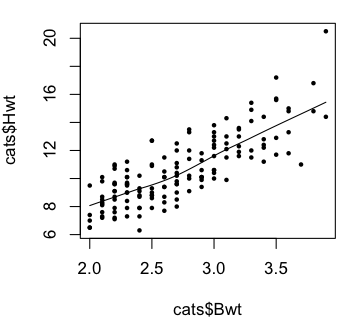The plots indicate we were on the right track with our linear model. revised 2016 February 15 | Table of Contents | Function Reference | Function Finder | R Project |